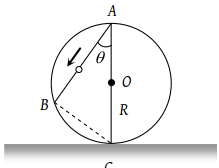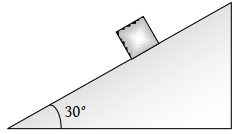A train is moving towards east and a car is along north, both with same speed. The observed direction of car to the passenger in the train is

(1) East-north direction

(2) West-north direction

(3) South-east direction

(4) None of these

Subtopic:  Relative Motion |
To view explanation, please take trial in the course below.
NEET 2022 - Target Batch - Aryan Raj Singh
To view explanation, please take trial in the course below.
NEET 2022 - Target Batch - Aryan Raj Singh

Difficulty Level:

A ball P is dropped vertically and another ball Q is thrown horizontally from the  same height and at the same time. If air resistance is neglected, then

(1) Ball P reaches the ground first

(2) Ball Q reaches the ground first

(3) Both reach the ground at the same time

(4) The respective masses of the two balls will decide the time

Subtopic:  Projectile Motion |
To view explanation, please take trial in the course below.
NEET 2022 - Target Batch - Aryan Raj Singh
To view explanation, please take trial in the course below.
NEET 2022 - Target Batch - Aryan Raj Singh

Difficulty Level:

A frictionless wire AB is fixed on a sphere of radius R. A very small spherical ball slips on this wire. The time taken by this ball to slip from A to B is(1) $\frac{2\sqrt{gR}}{g\mathrm{cos}\theta }$

(2) $2\sqrt{gR}.\frac{\mathrm{cos}\theta }{g}$

(3) $2\sqrt{\frac{R}{g}}$

(4) $\frac{gR}{\sqrt{g\mathrm{cos}\theta }}$

Subtopic:  Uniformly Accelerated Motion |
To view explanation, please take trial in the course below.
NEET 2022 - Target Batch - Aryan Raj Singh
To view explanation, please take trial in the course below.
NEET 2022 - Target Batch - Aryan Raj Singh

Difficulty Level:

A body is slipping from an inclined plane of height h and length l. If the angle of inclination is θ, the time taken by the body to come from the top to the bottom of this inclined plane is

(1) $\sqrt{\frac{2h}{g}}$

(2) $\sqrt{\frac{2l}{g}}$

(3) $\frac{1}{\mathrm{sin}\theta }\sqrt{\frac{2h}{g}}$

(4) $\mathrm{sin}\theta \sqrt{\frac{2h}{g}}$

Subtopic:  Acceleration |
To view explanation, please take trial in the course below.
NEET 2022 - Target Batch - Aryan Raj Singh
To view explanation, please take trial in the course below.
NEET 2022 - Target Batch - Aryan Raj Singh

Difficulty Level:

An aeroplane is moving with a velocity u. It drops a packet from a height h. The time t taken by the packet in reaching the ground will be

(1) $\sqrt{\left(\frac{2g}{h}\right)}$

(2) $\sqrt{\left(\frac{2u}{g}\right)}$

(3) $\sqrt{\left(\frac{h}{2g}\right)}$

(4) $\sqrt{\left(\frac{2h}{g}\right)}$

Subtopic:  Projectile Motion |
To view explanation, please take trial in the course below.
NEET 2022 - Target Batch - Aryan Raj Singh
To view explanation, please take trial in the course below.
NEET 2022 - Target Batch - Aryan Raj Singh

Difficulty Level:

An aeroplane is moving with horizontal velocity u at height h. The velocity of a packet dropped from it on the earth's surface will be (g is acceleration due to gravity)

(1) $\sqrt{{u}^{2}+2gh}$

(2) $\sqrt{2gh}$

(3) 2 gh

(4) $\sqrt{{u}^{2}-2gh}$

Subtopic:  Projectile Motion |
To view explanation, please take trial in the course below.
NEET 2022 - Target Batch - Aryan Raj Singh
To view explanation, please take trial in the course below.
NEET 2022 - Target Batch - Aryan Raj Singh

Difficulty Level:

A bullet is fired with a speed of $1000\text{\hspace{0.17em}\hspace{0.17em}}m/\mathrm{sec}$ in order to hit a target 100 m away. If $g=10\text{\hspace{0.17em}\hspace{0.17em}}m/{s}^{2}$, the gun should be aimed:

(1) Directly towards the target

(2) 5 cm above the target

(3) 10 cm above the target

(4) 15 cm above the target

Subtopic:  Projectile Motion |
To view explanation, please take trial in the course below.
NEET 2022 - Target Batch - Aryan Raj Singh
To view explanation, please take trial in the course below.
NEET 2022 - Target Batch - Aryan Raj Singh

Difficulty Level:

A body sliding on a smooth inclined plane requires 4 seconds to reach the bottom starting from rest at the top. How much time does it take to cover one-fourth distance starting from the rest at the top?

(1) 1 s

(2) 2 s

(3) 4 s

(4) 16 s

Subtopic:  Uniformly Accelerated Motion |
To view explanation, please take trial in the course below.
NEET 2022 - Target Batch - Aryan Raj Singh
To view explanation, please take trial in the course below.
NEET 2022 - Target Batch - Aryan Raj Singh

Difficulty Level:

The time taken by a block of wood (initially at rest) to slide down a smooth inclined plane 9.8 m long (angle of inclination is $30°$) is:(1) $\frac{1}{2}\mathrm{sec}$

(2) 2 sec

(3) 4 sec

(4) 1 sec

Subtopic:  Uniformly Accelerated Motion |
To view explanation, please take trial in the course below.
NEET 2022 - Target Batch - Aryan Raj Singh
To view explanation, please take trial in the course below.
NEET 2022 - Target Batch - Aryan Raj Singh

Difficulty Level:

Read the assertion and reason carefully to mark the correct option out of the options given below:

(1) If both assertion and reason are true and the reason is the correct explanation of the assertion.

(2) If both assertion and reason are true but reason is not the correct explanation of the assertion.

(3) If assertion is true but reason is false.

(4) If the assertion and reason both are false.

(5) If assertion is false but reason is true.

Assertion : Rocket in flight is not an illustration of projectile.

Reason : Rocket takes flight due to combustion of fuel and does not move under the gravity effect alone.

1. If both the assertion and the reason are true and the reason is a correct explanation of the assertion
2. If both the assertion and reason are true but the reason is not a correct explanation of the assertion
3. If the assertion is true but the reason is false
4. If both the assertion and reason are false
Subtopic:  Projectile Motion |
To view explanation, please take trial in the course below.
NEET 2022 - Target Batch - Aryan Raj Singh
To view explanation, please take trial in the course below.
NEET 2022 - Target Batch - Aryan Raj Singh

Difficulty Level: